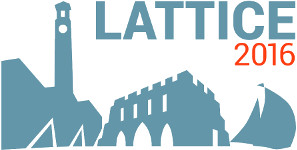#The 34th International Symposium on Lattice Field Theory (Lattice 2016)

Jul 24 – 30, 2016
Highfield Campus, University of Southampton
Europe/London timezone

## Discrete $\beta$-function of the SU(3) gauge theory with 10 massless domain-wall fermions

Jul 26, 2016, 3:20 PM
20m
Building 67 Room 1027 (Highfield Campus, University of Southampton)

### Building 67 Room 1027

#### Highfield Campus, University of Southampton

Highfield Campus, Southampton SO17 1BJ, UK
Talk Physics Beyond the Standard Model

### Speaker

Prof. Ting-Wai Chiu (National Taiwan University)

### Description

I present the updated results of the discrete $\beta$-function of the $SU(3)$ gauge theory with $N_f=10$ massless optimal domain-wall fermions in the fundamental representation. The renormalized coupling is obtained by the finite-volume gradient flow scheme on $L^4$ lattices, for seven lattice sizes: $L/a = 8, 10, 12, 16, 20, 24, 32$; and each with $11 - 17$ different lattice spacings parametrized by $6/g_0^2$. The discrete $\beta$-function is extrapolated to the continuum limit using four lattice pairs $(L, 2L)/a =(8, 16), (10, 20), (12, 24)$ and $(16,32)$. This provides stronger evidence of the infrared fixed point at $g_c^2 \simeq 7$, which was first reported in arXiv:1603.08854, based on the continuum extrapolation of the discrete $\beta$-function obtained with three lattice pairs.

### Primary author

Prof. Ting-Wai Chiu (National Taiwan University)

### Presentation materials

There are no materials yet.